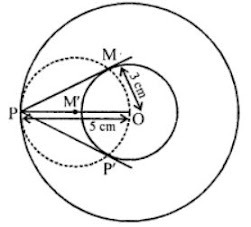# RD Sharma Solutions Chapter 11 Constructions Exercise 11.3 Class 10 Maths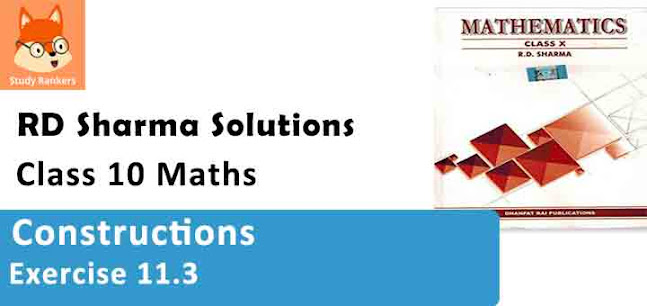Chapter Name RD Sharma Chapter 11 Constructions Book Name RD Sharma Mathematics for Class 10 Other Exercises Exercise 11.1Exercise 11.2Exercise 11.3 Related Study NCERT Solutions for Class 10 Maths

### Exercise 11.3 Solutions

1. Draw a circle of radius 6 cm. From a point 10 cm away from its centre, construct the pair of tangents to the circle and measure their lengths.

Solution

Steps of construction :

1. Draw a circle with O centre and 6 cm radius.
2. Take a point P, 10 cm away from the centre O.
3. Join PO and bisect it at M.
4. With centre M and diameter PO, draw a circle intersecting the given circle at T and S.
5. Join PT and PS.
Then PT and PS are the required tangents.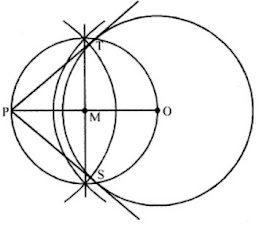2. Draw a circle of radius 3 cm. Take two points P and Q on one of its extended diameter each at a distance of 7 cm from its centre. Draw tangents to the circle from these two points P and Q.

Solution

Steps of construction :

1. Draw a circle with centre O and radius 3 cm.
2. Draw a diameter and produce it to both sides.
3. Take two points P and Q on this diameter with a distance of 7 cm each from the centre O.
4. Bisect PO at M and QO at N
5. With centres M and N, draw circle on PO and QO as diameter which intersect the given circle at S, T and S', T' respectively.
6. Join PS, PT, QS' and QT'.
Then PS, PT, QS' and QT' are the required tangents to the given circle.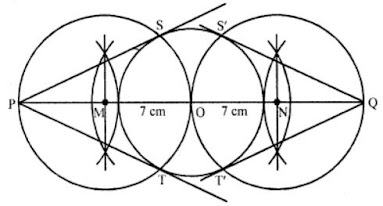3. Draw a line segment AB of length 8 cm. Taking A as centre, draw a circle of radius 4 cm and taking B as centre, draw another circle of radius 3 cm. Construct tangents to each circle from the centre of the other circle.

Solution

Steps of construction :

1. Draw a line segment AB = 8 cm.
2. With centre A and radius 4 cm and with centre B and radius 3 cm, circles are drawn.
3. Bisect AB at M.
4. With centre M and diameter AB, draw a circle which intersects the two circles at S', T' and S, T respectively.
5. Join AS, AT, BS' and BT'.

Then AS, AT, BS' and BT' are the required tangent.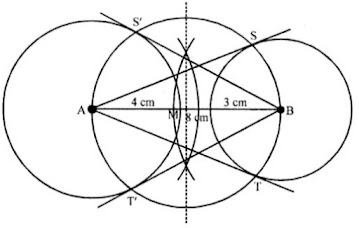4. Draw two tangents to a circle of radius 3.5 cm from a point P at a distance of 6.2 cm from its centre.

Solution

Steps of construction :

1. Draw a circle with centre O and radius 3.5 cm
2. Take a point P which is 6.2 cm from O.
3. Bisect PO at M and draw a circle with centre M and diameter OP which intersects the given circle at T and S respectively.
4. Join PT and PS.
PT and PS are the required tangents to circle.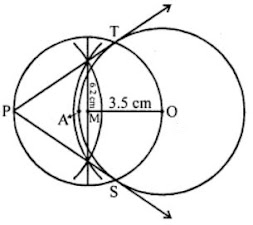5. Draw a pair of tangents to a circle of radius 4.5 cm, which are inclined to each other at an angle of 45°.

Solution

Steps of construction :
Angle at the centre 180° – 45° = 135°
(i) Draw a circle with centre O and radius 4.5 cm.
(ii) At O, draw an angle ∠TOS = 135°
(iii) At T and S draw perpendicular which meet each other at P.
PT and PS are the tangents which inclined each other 45°.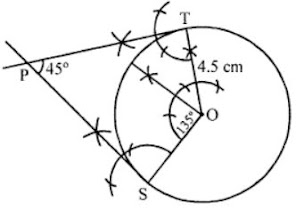6. Draw a right triangle ABC in which AB = 6 cm, BC = 8 cm and ∠B = 90°. Draw BD perpendicular from B on AC and draw a circle passing through the points B, C and D. Construct tangents from A to this circle.

Solution

Steps of Construction :

1. Draw a line segment BC = 8 cm
2. From B draw an angle of 90°
3. Draw an arc BA = 6cm cutting the angle at A.
4. Join AC.
5. ΔABC is the required A.
6. Draw ⊥ bisector of BC cutting BC at M.
7. Take M as centre and BM as radius, draw a circle.
8. Take A as centre and AB as radius draw an arc cutting the circle at E. Join AE.
AB and AE are the required tangents.
Justification :
∠ABC = 90° (Given)
Since, OB is a radius of the circle.
∴ AB is a tangent to the circle.
Also, AE is a tangent to the circle.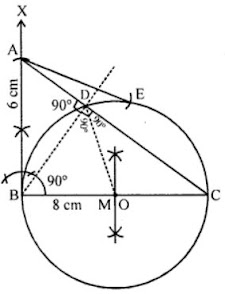7. Draw two concentric circles of radii 3 cm and 5 cm. Construct a tangent to the smaller circle from a point on the larger circle. Also, measure its length.

Solution

Given, two concentric circles of radii 3 cm and 5 cm with centre O. We have to draw pair of tangents from point P on outer circle to the other.
Steps of construction :

1. Draw two concentric circles with centre O and radii 3 cm and 5 cm.
2. Taking any point P on outer circle. Join OP.
3. Bisect OP, let M' be the mid-point of OP.
4. Taking M' as centre and OM' as radius draw a circle dotted which cuts the inner circle as M and P'.
5. Join PM and PP’. Thus, PM and PP’ are the required tangents.
6. On measuring PM and PP', we find that PM = PP' = 4 cm.
Actual calculation:
In right angle ΔOMP, ∠PMO = 90°
∴ PM2 = OP2 – OM2
[by Pythagoras theorem i.e. (hypotenuse)2 = (base)2 + (perpendicular)2]
⇒ PM2 = (5)2 – (3)2 = 25 – 9 = 16
⇒ PM = 4 cm
Hence, the length of both tangents is 4 cm.